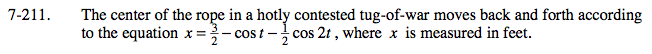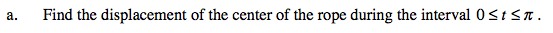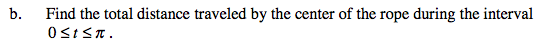Home > CALC > Chapter Ch7 > Lesson 7.4.4 > Problem7-211

7-211.
1. The center of the rope in a hotly contested tug-of-war moves back and forth according to the equation x =− cos tcos 2t, where x is measured in feet. Homework Help ✎

1. Find the displacement of the center of the rope during the interval 0 ≤ tπ.

2. Find the total distance traveled by the center of the rope during the interval 0 ≤ tπ.displacement over time = position at end – position at startIf x(t) was guaranteed to be strictly positive throughout the interval, then the total distance would equal the displacement.
But you were not given this guarantee, so you will have to investigate whether there is a direction change, or not.

Is there anywhere in which x ′(t) < 0 ?